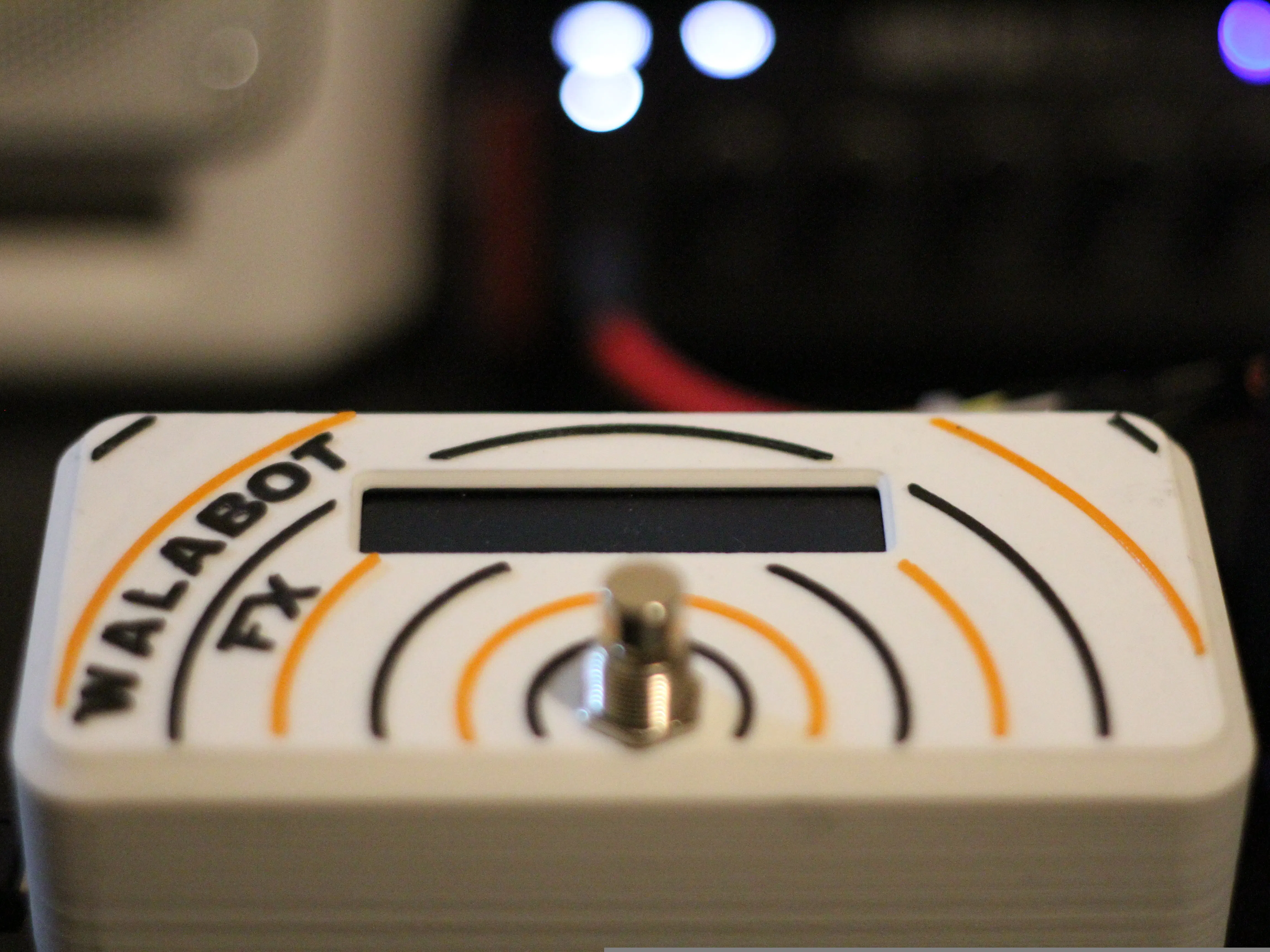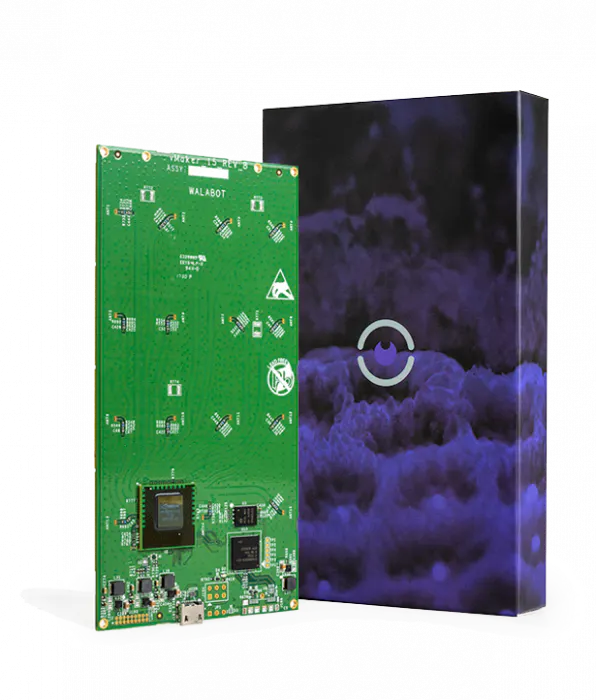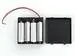Published © CC BY-NC-SA

Walabot FX - Guitar Effect Control

Control your favourite guitar effect using nothing but awesome guitar poses.

IntermediateFull instructions provided2 days2,876Things used in this project

Hardware componentsWalabot Creator
×1Raspberry Pi 3 Model B
×1
 Sunfounder LCD1602
×1
 SunFounder PCA9685 16 Channel 12 Bit PWM Servo Driver for Arduino and Raspberry Pi
×1
 Servos (Tower Pro MG996R)
×39V Battery Clip
×14xAA battery holder
×1AA Batteries
×1Jumper wires (generic)
×2
 DPDT Latching Action Foot Switch
×1
 Korg SDD3000-PDL
×1

Software apps and online servicesAutodesk Fusion 360Blynk

Hand tools and fabrication machines3D Printer (generic)Soldering iron (generic)

Custom parts and enclosures

Walabot FX Servo Bracket

Use this to attach the servo to your effect

Walabot FX Servo Pot Adapter

Use this adapter to connect your servos to your gutar effect controls

Schematics

Walabot FX Wiring Diagram

Use this with jumper wires to hook up your Walabot FX

Code

GuitarEffectCLI.py

Python
Use this to interface your walabot with servos
from __future__ import print_function
from sys import platform
from os import system
from blynkapi import Blynk
import WalabotAPI
import time
import RPi.GPIO as GPIO

#set up GPIO using Board Numbering
GPIO.setmode(GPIO.BOARD)
GPIO.setup(18, GPIO.IN, pull_up_down = GPIO.PUD_UP)

#blynk auth token
auth_token = "auth_token_here"

# Import the PCA9685 module for servo control.

#import LCD module from location
from imp import load_source
LCD1602 = load_source('LCD1602', '/usr/share/sunfounder/Python/LCD1602.py')

# Initialise the PCA9685 using the default address (0x40).

# blynk objects
defaults = Blynk(auth_token, pin = "V9")
start_button = Blynk(auth_token, pin = "V3")
Rmax = Blynk(auth_token, pin = "V0")
Rmin = Blynk(auth_token, pin = "V1")
Rres = Blynk(auth_token, pin = "V2")

ThetaMax = Blynk(auth_token, pin = "V4")
ThetaRes = Blynk(auth_token, pin = "V5")

PhiMax = Blynk(auth_token, pin = "V6")
PhiRes = Blynk(auth_token, pin = "V7")

Threshold = Blynk(auth_token, pin = "V8")

ServoMin = Blynk(auth_token, pin = "V10")
ServoMax = Blynk(auth_token, pin = "V11")

def LCDsetup():
LCD1602.init(0x27, 1)   # init(slave address, background light)

def numMap(x, in_min, in_max, out_min, out_max):
""" used for mapping the walabot readings to servo position """
return int((x- in_min) * (out_max - out_min) / (in_max - in_min) + out_min)

# use this for rounding up the raw data to the assigned value
def myRound(x, base=2):
return int(base * round(float(x)/base))

#extracts the number form the returned blynk string
def numberExtract(val):
val = str(val)
return int(filter(str.isdigit, val))

# Set frequency to 60hz, good for servos.
pwm.set_pwm_freq(60)

# Configure min and max servo pulse lengths defaults
SERVO_MIN = 175 # Min pulse length out of 4096
SERVO_MAX = 575  # Max pulse length out of 4096

# walabot default values
R_MAX = 60
R_MIN = 20
R_RES = 5

THETA_MAX = 20
THETA_RES = 5

PHI_MAX = 20
PHI_RES = 5

THRESHOLD = 1

# variables for blynk switching
on = "[u'1']"

class Walabot:

def __init__(self):
self.wlbt = WalabotAPI
self.wlbt.Init()
self.wlbt.SetSettingsFolder()
self.isConnected = False
self.isTargets = False
self.distance = lambda t: (t.xPosCm**2+t.yPosCm**2+t.zPosCm**2) ** 0.5

def blynkConfig(self):
if str(load_defaults) == on:
SERVO_MAX = ServoMax.get_val()
SERVO_MAX = numberExtract(SERVO_MAX)
print("Servo Max =", SERVO_MAX)

SERVO_MIN = ServoMin.get_val()
SERVO_MIN = numberExtract(SERVO_MIN)
print("Servo MIN =", SERVO_MIN)

R_MAX = Rmax.get_val()
R_MAX = numberExtract(R_MAX)
print("R max =", R_MAX)

R_MIN = Rmin.get_val()
R_MIN = numberExtract(R_MIN)
print("R Min =", R_MIN)

R_RES = Rres.get_val()
R_RES = numberExtract(R_RES)
print("R Res =", R_RES)

THETA_MAX = ThetaMax.get_val()
THETA_MAX = numberExtract(THETA_MAX)
print("Theta Max =", THETA_MAX)

THETA_RES = ThetaRes.get_val()
THETA_RES = numberExtract(THETA_RES)
print("Theta Res =", THETA_RES)

PHI_MAX = PhiMax.get_val()
PHI_MAX = numberExtract(PHI_MAX)
print("Phi Max =", PHI_MAX)

PHI_RES = PhiRes.get_val()
PHI_RES = numberExtract(PHI_RES)
print("Phi Res =", PHI_RES)

THRESHOLD = Threshold.get_val()
THRESHOLD = numberExtract(THRESHOLD)
print("Threshold =", THRESHOLD)

else: # if nothing from blynk app, load defaults
SERVO_MIN = 175 # Min pulse length out of 4096
SERVO_MAX = 575  # Max pulse length out of 4096

# walabot default values
R_MAX = 60
R_MIN = 20
R_RES = 5

THETA_MAX = 20
THETA_RES = 5

PHI_MAX = 20
PHI_RES = 5

THRESHOLD = 1

def connect(self):
try:
self.wlbt.ConnectAny()
self.isConnected = True
self.wlbt.SetProfile(self.wlbt.PROF_SENSOR)
#self.wlbt.SetProfile(self.wlbt.PROF_TRACKER)
#self.wlbt.SetDynamicImageFilter(self.wlbt.FILTER_TYPE_MTI)
self.wlbt.SetDynamicImageFilter(self.wlbt.FILTER_TYPE_NONE)
#self.wlbt.SetDynamicImageFilter(self.wlbt.FILTER_TYPE_DERIVATIVE)
self.wlbt.SetArenaTheta(-THETA_MAX, THETA_MAX, THETA_RES)
self.wlbt.SetArenaPhi(-PHI_MAX, PHI_MAX, PHI_RES)
self.wlbt.SetArenaR(R_MIN, R_MAX, R_RES)
self.wlbt.SetThreshold(THRESHOLD)

except self.wlbt.WalabotError as err:
if err.code != 19:  # 'WALABOT_INSTRUMENT_NOT_FOUND'
raise err

def start(self):
self.wlbt.Start()

def calibrate(self):
self.wlbt.StartCalibration()

def get_targets(self):
self.wlbt.Trigger()
#return self.wlbt.GetTrackerTargets()
return self.wlbt.GetSensorTargets()

def stop(self):
self.wlbt.Stop()

def disconnect(self):
self.wlbt.Disconnect()
self.wlbt.Clean()

def main():
flag = True
check = ""
LCDsetup()
while flag:
LCD1602.write(0, 0, 'Guitar        ')
LCD1602.write(0, 1, 'Effect Control')
time.sleep(2)
LCD1602.write(0, 0, 'Press Start to  ')
LCD1602.write(0, 1, 'begin           ')
time.sleep(2)
if (str(check) == on):
flag = False
else:
check = start_button.get_val()  #check for blynk start button press

if (GPIO.input(18) == 0): #check footswitch
flag = False

LCD1602.write(0, 0, "OK! let's Do it ")
LCD1602.write(0, 1, '                ')

wlbt = Walabot()
wlbt.blynkConfig()
wlbt.connect()
LCD1602.clear()
if not wlbt.isConnected:
LCD1602.write(0, 0, 'Not Connected ')
else:
LCD1602.write(0, 0, 'Connected     ')
time.sleep(2)
wlbt.start()
wlbt.calibrate()
LCD1602.write(0, 0, 'Calibrating.....')
time.sleep(3)
LCD1602.write(0, 0, 'Starting Walabot')

appcheck = start_button.app_status()

flag = True # reset flag for main prog

while flag: # used to put effect in standby (effectively)
if (appcheck == True):
if (str(check) != on):
if (GPIO.input(18) != 0): #check footswitch
flag = False
else:
check = start_button.get_val()  #check for start button press
appcheck = start_button.app_status()

else:
if (GPIO.input(18) != 0): #check footswitch
flag = False

xval = 0
yval = 0
zval = 0

average = 1
delayTime = 0

targets = wlbt.get_targets()

if len(targets) > 0:
for j in range(average):

targets = wlbt.get_targets()
if len(targets) > 0:

print(len(targets))
targets = targets

print(str(targets.xPosCm))
xval += int(targets.xPosCm)
yval += int(targets.yPosCm)
zval += int(targets.zPosCm)
time.sleep(delayTime)
else:
print("no targets")

xval = xval/average

xval = numMap(xval, -60, 60, SERVO_MIN, SERVO_MAX)
xval = myRound(xval)
if xval < SERVO_MIN: # protect the servo
xval = SERVO_MIN
if xval > SERVO_MAX:
xval = SERVO_MAX
LCD1602.write(0, 0, 'x=' + str(xval) + '           ')
pwm.set_pwm(0, 0, xval)

yval = yval/average

yval = numMap(yval, -60, 60, SERVO_MIN, SERVO_MAX)
yval = myRound(yval)
if yval < SERVO_MIN: # protect the servo
yval = SERVO_MIN
if yval > SERVO_MAX:
yval = SERVO_MAX
LCD1602.write(0, 1, 'y=' + str(yval))
pwm.set_pwm(1, 0, yval)

zval = zval/average

zval = numMap(zval, R_MIN, R_MAX, SERVO_MIN, SERVO_MAX)
zval = myRound(zval)
if zval < SERVO_MIN: # protect the servo
zval = SERVO_MIN
if zval > SERVO_MAX:
zval = SERVO_MAX
LCD1602.write(8, 1, 'z=' + str(zval))
pwm.set_pwm(2, 0, zval)

else:
print("no targets")
LCD1602.write(0, 0, "Shutting Down   ")
LCD1602.write(0, 1, 'The Walabot     ')
time.sleep(3)
wlbt.stop()
wlbt.disconnect()

if __name__ == '__main__':
while True:
main()

guitareffect.sh

Powershell
use this to start your python code
#!/bin/sh
cd /home/pi
sudo python GuitarEffectCLI.py

rc.local

Powershell
add these to your rc.local file so the programs run automatically
#!/bin/sh -e
#
# rc.local
#
# This script is executed at the end of each multiuser runlevel.
# Make sure that the script will "exit 0" on success or any other
# value on error.
#
# In order to enable or disable this script just change the execution
# bits.
#
# By default this script does nothing.

# Print the IP address
_IP=\$(hostname -I) || true
if [ "\$_IP" ]; then
printf "My IP address is %s\n" "\$_IP"
fi

./blynk-library/linux/blynk --token="you token goes here" &
sleep 10
sudo /home/pi/guitareffect.sh &
exit 0

LCD Python Lib

Use this for the Sunfounder LCD

Credits

Dave Clarke

14 projects • 66 followers
Industrial Designer, Maker of Things, Lover of Phi and all around nice guy